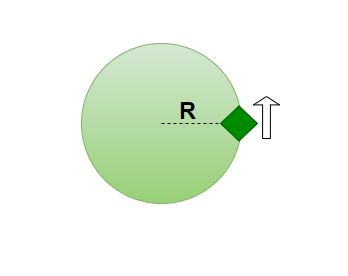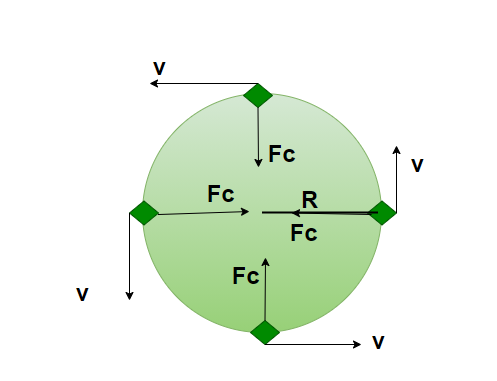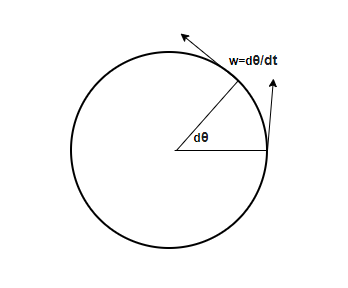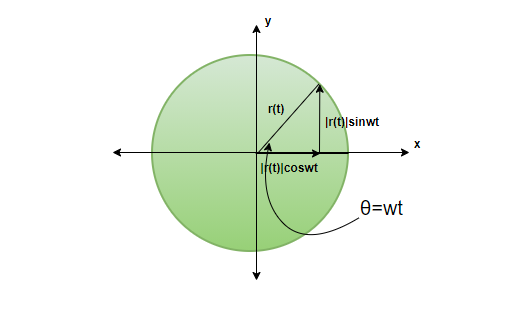# Uniform Circular Motion

• Last Updated : 07 May, 2021

Motion in a plane is when an object moves in two coordinates, say x, y or y, z, and so on. One of the examples of 2-D Motion is Projectile motion where the object moves in both horizontal and vertical directions. Uniform circular motion is another example of 2-dimensional motion, where the object moves with uniform speed in a circular motion while the velocity keeps on changing at every point since the direction of the velocity vector keeps on changing.

### What is Uniform Circular Motion?

Uniform Circular motion is the 2-dimensional motion in which the object moves with a uniform speed in a fixed circular direction but since the direction of the object keeps on changing at each and every point, the velocity keeps on changing as well, the direction at every point is the direction towards the tangent.When the object travels in a circular motion, at every point, some acceleration is experienced by the object, the acceleration acts towards the centre of the circle which makes the object move in that circle. The acceleration is known as Radial acceleration or Centripetal acceleration

In a uniform circular motion, the force acting towards the centre is called centripetal force and in order to balance that force, the force that acts outside the circle is known as centrifugal force.Note:

• The Centripetal force always acts towards the centre.
• The direction of Velocity is always tangent to the circle at all points.
• The acceleration vector will always be perpendicular to the velocity vector and hence, will always point towards the centre.
• The angular velocity is given as, w= v/r

where, w= angular velocity

v = magnitude of velocity

r = radius of the circle

• The magnitude of acceleration is given as, a=v2/r
• The value of angular acceleration is always zero in uniform circular motion since the angular velocity is constant.

### Angular displacement

In the circular path, angular displacement is the angle subtended by the position vector to the centre. The unit of the angular displacement is Radians. The formula for angular displacement is,

dθ = dS/r

Where,

dS = Linear displacement

r = radius of the circle.

### Angular Velocity

Angular velocity is defined just like linear velocity, that is, Angular velocity is the rate of change of angular displacement. The unit for angular velocity is Radian/sec. It is given as,w = dθ/dt (rad/sec)

The linear velocity is related to angular velocity as, v=wr.

### Angular Acceleration

The acceleration is defined as the rate of change of angular velocity (w), The unit for angular acceleration is given as Radian/sec2. The linear acceleration is related to angular acceleration as, al = αr

The angular acceleration is given as,

α = dw/dt

Where,

α = angular acceleration

dw= change in angular velocity

dt= change in time

### Centripetal Force

There is acceleration, therefore, there must be a force. The net force acting on the object in a uniform circular motion is inwards and is called centripetal force.

The Force acting towards the centre is given as,### Equation of motion for Uniform Circular Motion

The object moving in a uniform circular motion will have a certain position and speed at each point of time and hence, can be denoted by a position vector. Imagine that the particle has a position vectorWith an amplitude of P, The vector is travelling in both x and y coordinates hence, it will have components of both x and y-axis. In general, if the object is moving with a uniform angular frequency of ‘w’, at time ‘t’, it will have following values,

Position vector (P) ⇢X-Component of position vector ⇢Y- Component of position vector ⇢Where, w is given as,T= Time Period

Equation for Position Vector,The velocity vector can easily be obtained by differentiating the position vector w.r.t time,Similarly, the acceleration vector can be easily obtained by differentiating the velocity vector w.r.t. time,From the above equation, the relationship between acceleration vector and position vector can be easily calculated,### Sample Problems

Question 1: Give Practical examples of uniform circular motion.

Practical examples of Uniform Circular motion:

• The motion of the electrons in an atom around the nucleus.
• Wall of Death: The bike has a normal force acting towards the centre which makes it move in a circle.
• The Artificial satellite moving around the Earth is an example of uniform circular motion. The gravitational force from the centre of the earth exerted upon the satellite acts as the centripetal force for it.

Question 2: A cyclist is taking a turn with a speed of 5m/sec, if he doubles his speed, how will the force acting on his bicycle towards the centre will change?

Solution:

The centripetal force is given as,It is observed that F∝ v2

Therefore, if the velocity is doubled, the force acting on the bicycle will become 4 times than before.

Question 3: A plane is flying with a speed of 120 m/sec, it makes a turn to join a circular path levelling with the ground. What will be the radius of the circular path formed if the centripetal acceleration is equal to acceleration due to gravity.

Solution:

The centripetal acceleration is given as,

ac =v2/r

r= ac× v2

Let’s assume the acceleration due to gravity to be 10m/sec2

r= 10 × 120 × 120

r= 144000m

r= 144 km

Question 4: What is the device used for measuring the speed of rotation in electrical machines or in objects moving in uniform circular motors?

Tachometer is the device which is used to measure the speed of rotation in electrical machines or in objects moving in uniform circular motion.

Question 5: The property of conservation of energy is applied when an object moves in uniform circular motion. How?

The conservation of energy is a universal fact and this is properly applied when an object moves in uniform circular motion, when the object moves in uniform circular motion, the speed remains constant and hence, the kinetic energy remains constant. however, due to the constant change in velocity, the momentum keeps on changing.

Question 6: Suppose you are sitting in room and felt the need to increase the speed of the fan, you increased the regulator and the blades accelerated at 2 rad/sec2. It accelerated for 3 seconds and the final angular frequency of the blades became 7 rad/sec. What was the initial angular frequency?

Solution:

The formula for Angular velocity is,

α = Δw/ Δt

The time for which the blades accelerated= 3 seconds.

Acceleration = 2 rad/sec2

2 ={wf – wi}/ Δt

2 = {7 – wi} / 3

7- wi = 6

wi = 1 rad/sec

My Personal Notes arrow_drop_up